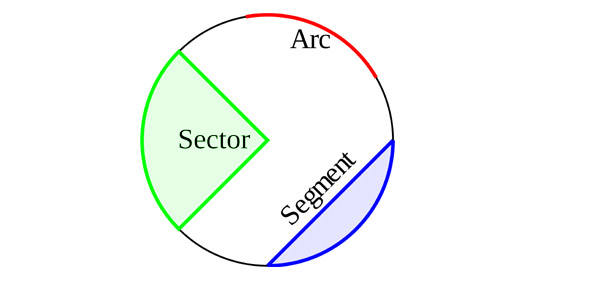# ACT 5-in-5 #1-5 Recap

8 QuestionsSettings.

Related Topics
• 1.
The expression a[b + (cd)] is equivalent to:
• A.

A. ab + ac – ad

• B.

B. ab + abc – ad

• C.

C. ab + ac – d

• D.

D. ab + c + d

• E.

E. ab + c – d

• 2.
For all x, (2x + 3)(4x – 5) = ?
• A.

• B.

• C.

• D.

• E.

• 3.

• A.

• B.

• C.

• D.

• E.

• 4.
• A.

• B.

15

• C.

• D.

35

• E.

45

• 5.
A circle in the standard (x, y) coordinate plane is tangent to the x-axis at 5 and tangent to the y-axis at 5.  Which of the following is an equation of the circle?
• A.

• B.

• C.

• D.

• E.

• 6.
• A.

441

• B.

467

• C.

515

• D.

655

• E.

675

• 7.
If A = BC, B = x, and C = yz, then AB = ?
• A.

Xyzx

• B.

Xyzy

• C.

Zxyz

• D.

Xyyz

• E.

Xyz

• 8.
• A.

X < 3

• B.

X < -1/3

• C.

X > 1/3

• D.

X > -1/3

• E.

X > -3